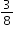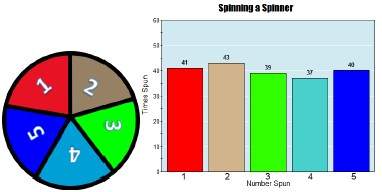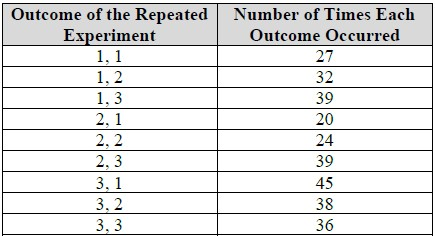# Standard #: MA.8.DP.2.3

This document was generated on CPALMS - www.cpalms.org

Solve real-world problems involving probabilities related to single or repeated experiments, including making predictions based on theoretical probability.

### Examples

Example: If Gabriella rolls a fair die 300 times, she can predict that she will roll a 3 approximately 50 times since the theoretical probability is.

Example: Sandra performs an experiment where she flips a coin three times. She finds the theoretical probability of landing on exactly one head as. If she performs this experiment 50 times (for a total of 150 flips), predict the number of repetitions of the experiment that will result in exactly one of the three flips landing on heads.

### Clarifications

Clarification 1: Instruction includes making connections to proportional relationships and representing probability as a fraction, percentage or decimal.

Clarification 2: Experiments to be repeated are limited to tossing a fair coin, rolling a fair die, picking a card randomly from a deck with replacement, picking marbles randomly from a bag with replacement and spinning a fair spinner.

Clarification 3: Repetition of experiments is limited to two times except for tossing a coin.

### General Information

Subject Area: Mathematics (B.E.S.T.)
Strand: Data Analysis and Probability
Status: State Board Approved

### Terms from the K-12 Glossary

• Theoretical Probability

### Vertical Alignment

Previous Benchmarks

Next Benchmarks

### Purpose and Instructional Strategies

In grade 7, students used a simulation of a simple experiment to find experimental probabilities and compare them to theoretical probabilities. In grade 8, students solve real-world problems involving probabilities related to single or repeated experiments, including making predictions based on theoretical probability. In high school, students will expand their knowledge to include more general experiments.
• Students should understand that results from an experiment do not always match the theoretical results, but if they do a large number of trials, they should be close.
• When determining experimental probabilities, students should understand that this may be done by performing a simple experiment more than once and also by performing a repeated experiment more than once.
• For example, to determine the experimental probability of tossing four heads in a row, the repeated experiment of tossing a coin four times will need to be performed many times (see also Benchmark Example 2).
• Instruction includes $P$($e$$v$$e$$n$$t$) notation.
• Instruction includes opportunities for students to run various numbers of trials to discover that the increased repetition of the experiment will bring the experimental probability closer to the theoretical. Use virtual simulations to quickly show higher and higher volumes of repetition that would be difficult to create with physical manipulatives (MTR.5.1).
• When comparing theoretical to experimental probability, it is important to not just compare the number of times the event occurs, but the probabilities themselves.

### Common Misconceptions or Errors

• Students may incorrectly assume the theoretical and experimental probabilities of the same experiment will always be the same. To address this misconception, provide multiple opportunities for students to experience simulations of different situations, with physical or virtual manipulatives, in order to find and compare the experimental and theoretical probabilities.
• Students may incorrectly expect to see every possible outcome occur during a simulation. While all may occur in a simulation, it is not certain to happen. Students may inadvertently let their own experience with an experiment affect their response.
• For example, during an experiment if a student never draws an ace from a standard deck of cards, this does not indicate it could never happen.
• Students may incorrectly believe the theoretical probability of an event is the proportion of times that event will actually occur.

### Strategies to Support Tiered Instruction

• Teacher reviews the root words theoretical (theory) and experimental (experiment) and discusses the difference between a theoretical probability and experimental probability. Teacher provides graphic organizer to keep as reference for root words.
• For example, experimental probabilities are from simulations whereas theoretical probabilities are from calculations.
• Teacher provides opportunities to discuss the difference between simulating simple experiments and simulating repeated experiments.
• For example, students could discuss tossing a coin 150 times as a simulation of the simple (single) experiment “tossing a coin” and tossing a coin 150 times as a simulation of the repeated experiment “tossing a coin three times.” In the first case, the simulation has 150 trials whereas the second simulation has 50 trials.
• Teacher sets up a simulation with several trials to work through and discuss what ‘we think’ should happen (Theoretical Probability) and what actually happens when the experiment is completed (Experimental Probability). Then, the teacher models how to find experimental probability, showing how the more trials that are done, the closer the results should get closer to the theoretical probability. Teacher provides instruction focused on color-coding when setting up a proportional relationship to ensure corresponding parts are placed in corresponding positions within the proportion.
• For example, if Jason choses a card from a standard deck of cards 104 times and replaces the card each time, what is a reasonable prediction on how many times he will choose a heart?• Instruction includes providing multiple opportunities to experience simulations of different situations, with physical or virtual manipulatives, in order to find and compare the experimental and theoretical probabilities.

The bar graph shows the results of spinning the spinner 200 times.• Part A. If the repeated experiment is to spin the spinner twice, predict how many times the event of landing on the same number twice will occur in 100 simulations of the repeated experiment.
• Part B. Using technology, perform a simulation of the experiment described in Part A.
• Part C. Compare the number of times you actually landed on the same number twice during the simulation to your prediction from Part A.

### Instructional Items

Instructional Item 1
If Jackson rolls a fair 6-sided die 200 times, what is a reasonable prediction on how many times he will roll a 4?

Instructional Item 2
A spinner is divided into three equal parts 1-3. The repeated experiment of spinning the spinner twice is simulated 300 times. A table of outcomes is shown.Based on the table, what is the experimental probability that the sum of the two outcomes is at least 4?

*The strategies, tasks and items included in the B1G-M are examples and should not be considered comprehensive.

#### Related Courses

 Course Number1111 Course Title222 1200400: Foundational Skills in Mathematics 9-12 (Specifically in versions: 2014 - 2015, 2015 - 2022, 2022 and beyond (current)) 1205050: M/J Accelerated Mathematics Grade 7 (Specifically in versions: 2014 - 2015, 2015 - 2020, 2020 - 2022, 2022 and beyond (current)) 1205070: M/J Grade 8 Pre-Algebra (Specifically in versions: 2014 - 2015, 2015 - 2022, 2022 and beyond (current)) 1204000: M/J Foundational Skills in Mathematics 6-8 (Specifically in versions: 2014 - 2015, 2015 - 2022, 2022 and beyond (current)) 7812030: Access M/J Grade 8 Pre-Algebra (Specifically in versions: 2014 - 2015, 2015 - 2018, 2018 - 2019, 2019 - 2022, 2022 and beyond (current))

#### Related Access Points

 Access Point Number Access Point Title MA.8.DP.2.AP.3 Compare actual results of an experiment with its theoretical probability (e.g., make a statement that describes the relationship between the actual results of an experiment with its theoretical probability [e.g., more, less, same, different, equal]).

#### Formative Assessments

 Name Description Coat Count Students are asked to design a simulation to generate frequencies for complex events. Probabilities Cubed Students are asked to estimate the frequency of an event given its probability and explain why an expected frequency might differ from an observed frequency.

#### Lesson Plans

 Name Description Independent Compound Probability During this lesson, students will use Punnett Squares to determine the probability of an offspring's characteristics. Understanding Probability of Compound Events This lesson uses guided teaching, small group activities, and student creations all-in-one! Students will be able to solve and create compound event word problems. They will also be able to identify what type of event is being used in a variety of word problems.

#### Original Student Tutorial

 Name Description Predicting Outcomes at the Carnival Learn how to use probability to predict expected outcomes at the Carnival in this interactive tutorial.

#### Perspectives Video: Experts

 Name Description Let's Make a Math Deal Should I keep my choice or switch?  Learn more about the origins and probability behind the Monty Hall door picking dilemma and how Game Theory and strategy effect the probability. Download the CPALMS Perspectives video student note taking guide. History of Probability and the Problem of Points What was the first question that started probability theory? Download the CPALMS Perspectives video student note taking guide.

#### Original Student Tutorial

 Name Description Predicting Outcomes at the Carnival: Learn how to use probability to predict expected outcomes at the Carnival in this interactive tutorial.

Printed On:9/26/2023 9:04:16 AM
Print Page | Close this window